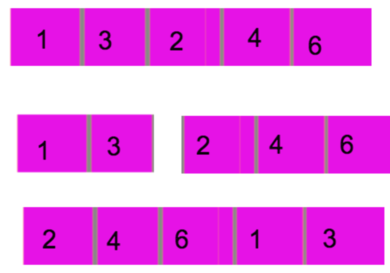HP and Splitting of Array
Tag(s):

## Advanced Data Structures, Algorithms, Data Structures, Fenwick (Binary Indexed) Trees, Fenwick Tree, Medium, Medium-hard

Problem
Editorial
Analytics

HP recently started learning about a property called inversion count. An inversion count of an array indicates how far from (or close to) being sorted the array is. If the array is already sorted, the inversion count is 0; if it's sorted in reverse order, the inversion count is maximal.

Formally speaking, two elements $A_{i}$ and $A_{j}$ form an inversion if $A_{i} > A_{j}$ and $i < j$. The inversion count is the number of pairs of indices $(i,j)$ such that $A_i$ and $A_j$ form an inversion.

You are given an array A with size N. Let's denote an i-rotation of this array as the array formed by removing the first i elements from the array and adding (appending) them to the end of the array in the same order. For example, the 2-rotation of the array $[1, 3, 2, 4, 6]$ is $[2, 4, 6, 1, 3]$.HP wants to know the inversion counts of i-rotations of array A. Compute this inversion count for each i ($1 \le i \le N$).

Constraints

• $1 \le T \le 10$
• $1 \le N \le 100,000$
• $1 \le A_{i} \le 1,000,000$ for each valid i
• all elements of the array A are unique

Input format

The first line of the input contains a single integer T — the number of test cases. Each test case consists of two lines.

The first line of each test case contains a single integer N denoting the size of the array.

The second line contains N space-separated integers $A_1,A_2,\dots,A_N$.

Output format

For each test case, print a single line containing N space-separated integers. The i-th of these integers should denote the inversion count of the i-rotation of A, for each $1 \le i \le N$.

SAMPLE INPUT
1
5
1 3 2 4 6
SAMPLE OUTPUT
5 5 7 5 1
Explanation

The array A is $[1, 3, 2, 4, 6]$. After moving the first element to the end, we get an array $[3, 2, 4, 6, 1]$. The inversions in this array are $(A_1,A_2)$, $(A_1,A_5)$, $(A_2,A_5)$, $(A_3,A_5)$ and $(A_4,A_5)$. Therefore, the inversion count of the 1-rotation of A is 5.

Time Limit: 1.0 sec(s) for each input file.
Memory Limit: 256 MB
Source Limit: 1024 KB
Marking Scheme: Marks are awarded when all the testcases pass.
Allowed Languages: Bash, C, C++, C++14, Clojure, C#, D, Erlang, F#, Go, Groovy, Haskell, Java, Java 8, JavaScript(Rhino), JavaScript(Node.js), Julia, Kotlin, Lisp, Lisp (SBCL), Lua, Objective-C, OCaml, Octave, Pascal, Perl, PHP, Python, Python 3, R(RScript), Racket, Ruby, Rust, Scala, Swift, Swift-4.1, TypeScript, Visual Basic

## CODE EDITOR

Initializing Code Editor...

## This Problem was Asked inChallenge Name

December Circuits '17

OTHER PROBLEMS OF THIS CHALLENGE
• Algorithms > Graphs
• Algorithms > Searching
• Data Structures > Arrays
• Algorithms > Dynamic Programming
• Math > Combinatorics